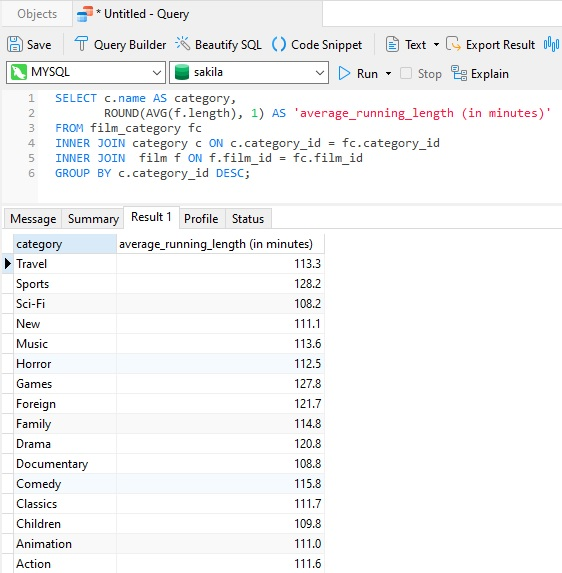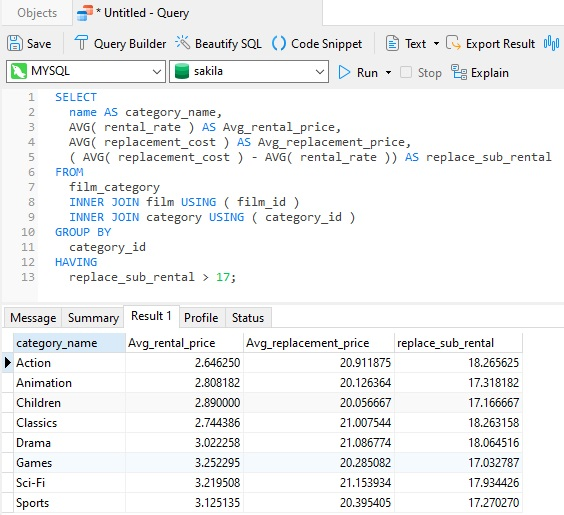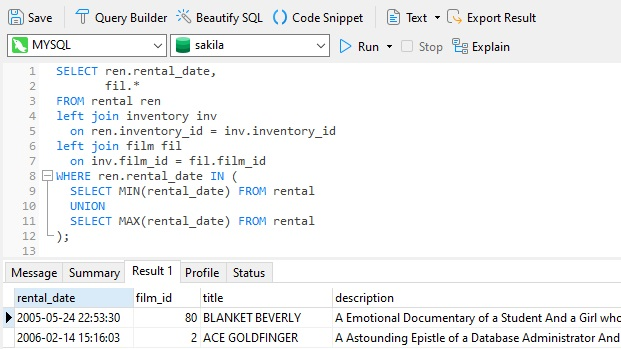# Navicat Blog

Some Useful MySQL Numeric Functions Feb 18, 2022 by Robert Gravelle

Back in May of 2021, we examined a few of SQL Server's Important SQL Server Functions. Now, it's time to turn our attention to MySQL to see what it offers us in terms of math and numeric functions. To see how they work in practice, we'll use them in queries that we'll run in Navicat 16 for MySQL.

# Comparing SQL Server and MySQL Functions

In the Important SQL Server Functions article, we reviewed the Abs, Round, Ceiling, and Floor functions. As it turns out, not only does MySQL also implement these functions, but with exactly the same names. Perhaps this is not surprising, as these are fundamental numeric functions across database products and programming languages.

Since the functions are the same in both DBMSes, there's no point in rehashing their use. Instead, we'll forge onwards and explore other useful numeric functions in MySQL.

# AVG

You probably already know what the Average, or Arithmetic Mean, is. You may even know that it is calculated by adding all of the value within a data set, and then dividing that result by the number of data points in the set. Hence, if we had five numbers such as 4, 5, 6, 5, 3, we would calculate their average as follows:

```(4 + 5 + 6 + 5 + 3) / 5 = 4.6
```

Simple enough to do with a small sample, but what happens when you have 10,000 rows? The answer, or course, is to use MySQL's built-in AVG function (identically named in SQL Server, by the way). All we need to do is provide it with a numeric expression and it returns its average value. Here's its simple syntax:

```AVG(expression)
```

Most of the time, you'll find yourself passing in a column name whose average you'd like to calculate. For example, here's a query that gives us the average running time of all the films in the Sakila Sample Database:The GROUP BY breaks up values by the category_id so that averages are based on each Film Type, i.e., "Action", "Drama", etc.

By passing the results of the AVG function to ROUND, we can omit some of the extra decimal places.

### A More Complex Example

The interesting thing about numeric functions is that they can be used as part of larger calculations. Case in point, here's a query that shows how many film categories in which the average difference between the film replacement cost and the rental rate larger than 17 dollars:To calculate the replacement cost, the average rental rate is subtracted from the average replacement cost. No need for temporary variables; just subtract the results of one function from the other:

```( AVG( replacement_cost ) - AVG( rental_rate ) ) AS replace_sub_rental
```

# MIN/MAX

Have you noticed that a lot of numeric functions have three letter names? Not sure why that is, but here are two related functions for calculating the minimum and maximum values of a set. Again, the most typical usage is to pass a column name to the function. The following query selects film details for the first and last rentals, according to the rental_date column. As such, it is passed to both the MIN and MAX functions:Mixing aggregate functions and scalar data can be problematic, so the MIN and MAX rental_dates are fetched within a subquery for comparison to those of each Film table row.

# Conclusion

This blog presented a few useful numeric functions in MySQL, including AVG, MIN, and MAX using Navicat 16 for MySQL as our database client. Speaking of which, if you'd like to give Navicat 16 for MySQL a test drive, you can download a 14 day trial here.

Blog Archives
Share
• Key Topics• Products• Partners